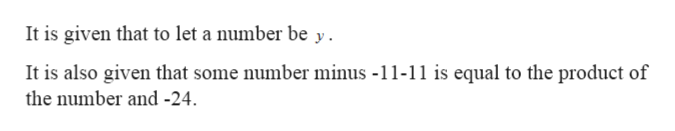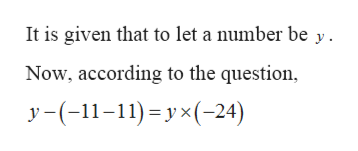# Translate each English phrase in the following problem into an algebraic expression and set up the related equation. Let y be the unknown number.Some number minus −11−11 is equal to the product of the number and −24.Step 1 of 3 :  Translate "Some number minus −11".

Question
9 views

Translate each English phrase in the following problem into an algebraic expression and set up the related equation. Let y be the unknown number.

Some number minus −11−11 is equal to the product of the number and −24.

Step 1 of 3 :

Translate "Some number minus −11".

check_circle

Step 1

Given: -help_outlineImage TranscriptioncloseIt is given that to let a number be y It is also given that some number minus -11-11 is equal to the product of the number and -24 fullscreen
Step 2

To find: -

Step 3

Calculation...help_outlineImage TranscriptioncloseIt is given that to let a number be y Now, according to the question y-(-1-11)y-24) fullscreen

### Want to see the full answer?

See Solution

#### Want to see this answer and more?

Solutions are written by subject experts who are available 24/7. Questions are typically answered within 1 hour.*

See Solution
*Response times may vary by subject and question.
Tagged in

### Other